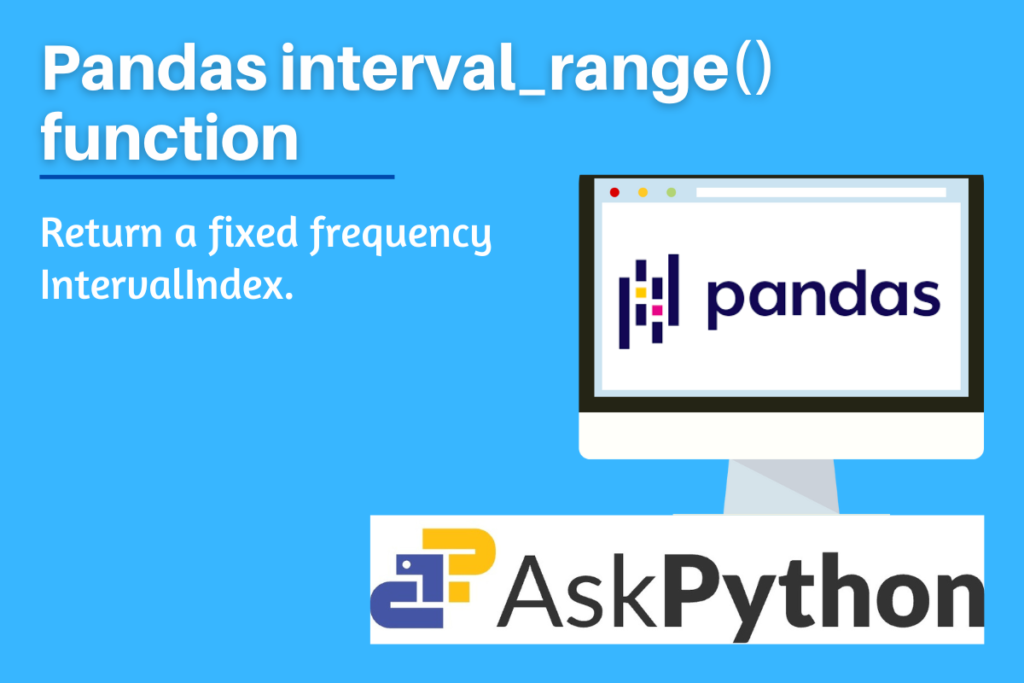# Pandas interval_range – Return a fixed frequency IntervalIndexLet’s attempt to comprehend the Pandas interval_range() function, one of the general techniques in the Pandas package, in this post. Data processing and interpretation are done with the help of the Python module Pandas. It is meant to refer to “Panel Data” and “Python Data Analysis” both as “Pandas”. It is an open-source package that works great with statistical datasets as well as vast tables with complex data. Hence Pandas package is a great tool for data scientists and data analysts.

The function discussed in this article is one of the functions used to return a fixed frequency set of values as an output. Let us understand its use cases, syntax, and its implementation in Python programming language.

## Why is Pandas interval_range() used?

This function is used to return an IntervalIndex with a fixed frequency. An index of Interval objects that are all closed on the same side is represented by IntervalIndex. Exact three of the four parameters start, end, periods, and freq must be provided. The resultant IntervalIndex will include linearly spaced entries between start and end, inclusively, if the frequency is left off.

Also, check out the timedelta_range() function and period_range() function of the Pandas package. They are very similar to the function we are discussing. The only difference is that it returns a fixed frequency in TimedeltaIndex format and PeriodIndex respectively.

## Syntax of Pandas interval_range()

```pandas.interval_range(start=None, end=None, periods=None, freq=None, name=None, closed='right')
```
• start: numeric or datetime-like, default is set to None
• For producing intervals, left bound.
• end: numeric or datetime-like, default is set to None
• For producing intervals, right bound.
• periods: integer, default is set to None
• The number of periods to produce.
• freq: numeric, string, or DateOffset, default is set to None
• the period of each interval. Must match the start and end types, for example, 2 for numeric or ‘5H’ for DateTime-like. Numeric defaults are 1 and DateTime-like defaults are ‘D’.
• name: string, default is set to None, Optional
• Name of the resulting IntervalIndex.
• closed: {‘left’, ‘right’, ‘both’, ‘neither’}, default is set to ‘right’, Optional
• either the left, right, both, or neither side of the intervals are closed.

## Implementing Pandas interval_range()

Before beginning the methods, be sure to load the pandas package into your IDE. Run the following line of code in your IDE to accomplish this.

```import pandas as pd
```

### Example 1: Passing only start and end parameters

```#numeric input
x = pd.interval_range(start=1, end=5)
print(x)

#timestamp input
y = pd.interval_range(start=pd.Timestamp('2000-01-01'), end=pd.Timestamp('2000-01-04'))
print(y)
```

Output

Note that in the above example, only the start and end parameters are passed, and no frequency or periods are assigned, hence all the values between the start value and the end value are present in the output.

### Example 2: Passing other parameters

```x = pd.interval_range(start=1, end=10, periods=3)
print(x)

y = pd.interval_range(start=pd.Timestamp('2000-01-01'), periods=3, freq='W')
print(y)

z = pd.interval_range(start=1, end=15, freq=3, name='Output')
print(z)
```

Output